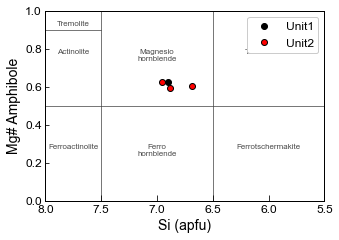This page was generated from docs/Examples/Amphibole/Amphibole_Classification_Diagrams.ipynb. Interactive online version:.

# Amphibole Classification Diagrams

:

# Load in some import Python things, and Thermobar
import numpy as np
import pandas as pd
import matplotlib.pyplot as plt
import Thermobar as pt
pd.options.display.max_columns = None


## You need to install Thermobar once on your machine, if you haven’t done this yet, uncomment the line below (remove the #)

:

#!pip install Thermobar

:

# Set some plotting parameters
plt.rcParams["font.family"] = 'arial'
plt.rcParams["font.size"] =12
plt.rcParams["mathtext.default"] = "regular"
plt.rcParams["mathtext.fontset"] = "dejavusans"
plt.rcParams['patch.linewidth'] = 1
plt.rcParams['axes.linewidth'] = 1
plt.rcParams["xtick.direction"] = "in"
plt.rcParams["ytick.direction"] = "in"
plt.rcParams["ytick.direction"] = "in"
plt.rcParams["xtick.major.size"] = 6 # Sets length of ticks
plt.rcParams["ytick.major.size"] = 4 # Sets length of ticks
plt.rcParams["ytick.labelsize"] = 12 # Sets size of numbers on tick marks
plt.rcParams["xtick.labelsize"] = 12 # Sets size of numbers on tick marks
plt.rcParams["axes.titlesize"] = 14 # Overall title
plt.rcParams["axes.labelsize"] = 14 # Axes labels


### Import amphiboles and associated liquids

• These amphiboles are from 2 units, unit 1 and unit 2

:

out=pt.import_excel('Amphibole_Liquids.xlsx', sheet_name="Amp_only_for_plotting")
my_input=out['my_input']
Amps=out['Amps']

:

## Check everything loaded in right, e.g., check no columns filled with zeros that should have data

SiO2_Amp TiO2_Amp Al2O3_Amp FeOt_Amp MnO_Amp MgO_Amp CaO_Amp Na2O_Amp K2O_Amp Cr2O3_Amp F_Amp Cl_Amp Sample_ID_Amp
0 42.43 2.50 12.97 7.80 0.09 15.56 11.21 2.41 1.61 0.31 0 0 Unit1
1 41.19 2.62 12.25 9.44 0.11 15.67 11.54 2.44 1.40 0.10 0 0 Unit1
2 45.69 1.44 9.64 13.37 0.21 14.57 10.72 1.76 0.23 0.00 0 0 Unit1
3 45.56 1.43 10.40 12.27 0.21 15.15 11.03 1.89 0.25 0.00 0 0 Unit1
4 45.65 1.55 10.78 13.30 0.21 14.21 10.81 1.89 0.27 0.00 0 0 Unit1

### Once we’ve loaded the data in, we can use the loc function to extract separate dataframes for unit 1 and unit2

:

Amps_Unit1=Amps.loc[Amps['Sample_ID_Amp']=="Unit1"]
Amps_Unit2=Amps.loc[Amps['Sample_ID_Amp']=="Unit2"]


### This function makes the plot automatically

• Here, we are plotting the amphiboles on Fig. 3 (bottom) from Leake, which is for Ca_B<1.5, and Na_A + K_A <0.5, CaA < 0.50

• The option “site_check=True” means that it only plots amphibles which fit these criteria, and the printed message tells you how many were excluded. If you want to plot ones outside this Ca_B and Na_A and K_A range anyway, select site_check=False.

• This is not as customizable as the bottom option, where we show how to construct the plot yourself, but provides a good first look.

:

fig=pt.plot_amp_class_Leake(amp_comps=Amps, fontsize=8, color=[0.3, 0.3, 0.3],
linewidth=0.5, lower_text=0.3, upper_text=0.7, text_labels=True, site_check=True,
plots="Ca_Amphiboles", marker='.k')

0 amphiboles have Ca_B<1.5, so arent shown on this plot
0 amphiboles have Ca_A>=0.5, so arent shown on this plot
17 amphiboles have Na_A+K_A>0.5, so arent shown on this plot### Making a more customizable plot, e.g., plotting different units as different colours

• Here, we are plotting the amphiboles on Fig. 3a from Leake, which is for Ca_B<1.5, and Na_A + K_A <0.5

• By default, this code will plot all amphiboles, some of which may not actually lie on this diagram.

:

fig, (ax1) = plt.subplots(1, figsize=(5, 3.5), sharey=True)

linewidth=0.5, lower_text=0.3, upper_text=0.8, text_labels=True)

# Now calculate the amphibole components
cat_23ox_Unit1=pt.calculate_Leake_Diagram_Class(amp_comps=Amps_Unit1)
cat_23ox_Unit2=pt.calculate_Leake_Diagram_Class(amp_comps=Amps_Unit2)

# You only want the ones where "Diagram" = Fig. 3 - bottom - LHS,
#Lets use Loc to find these rows
cat_23ox_Unit1_Correct=cat_23ox_Unit1.loc[
cat_23ox_Unit1['Diagram']=="Fig. 3 - bottom - LHS"]
cat_23ox_Unit2_Correct=cat_23ox_Unit2.loc[
cat_23ox_Unit2['Diagram']=="Fig. 3 - bottom - LHS"]

# Now add these components to the axis, you can change symbol size, plot multiple amphioble populations in different colors.
ax1.plot(cat_23ox_Unit1_Correct['Si_Amp_cat_23ox'],
cat_23ox_Unit1_Correct['Mgno_Amp'], 'ok', label='Unit1')
ax1.plot(cat_23ox_Unit2_Correct['Si_Amp_cat_23ox'],
cat_23ox_Unit2_Correct['Mgno_Amp'], 'ok', mfc='red', label='Unit2')

# Now reverse the x axis to match the common way of showing this in the literature
ax1.invert_xaxis()

ax1.set_ylabel('Mg# Amphibole')
ax1.set_xlabel('Si (apfu)')[ ]: